# Per Capita GDP by EU and US State, Second Quarter 2020

Oct 26, 2020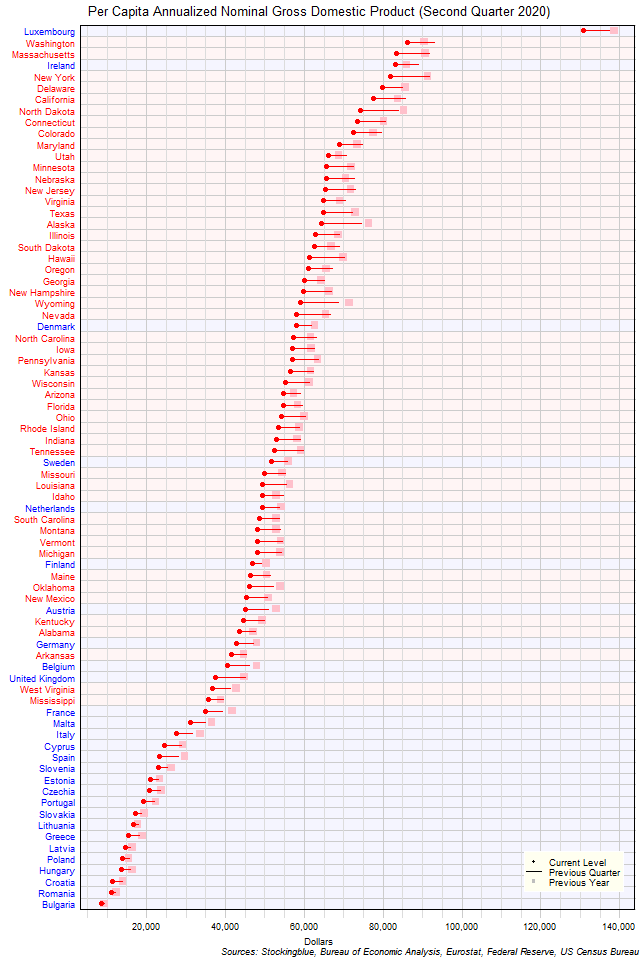The chart above shows the per capita annualized nominal gross domestic product (GDP) in each EU and US state as of the second quarter of 2020 in dollars, the change from the previous quarter, and the GDP one year prior.  Every single state's per capita GDP contracted over the past quarter and the past year.

# Per Capita GDP by EU State, Second Quarter 2020

Oct 23, 2020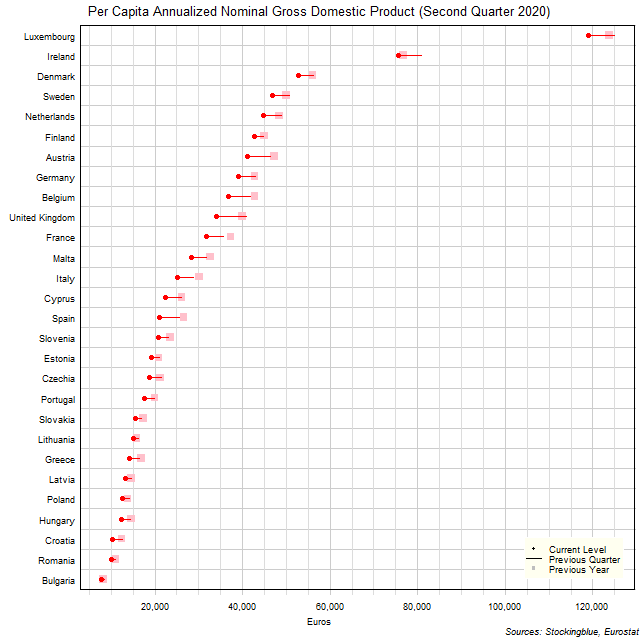The chart above shows the per capita annualized nominal gross domestic product (GDP) in each EU state as of the second quarter of 2020 in euros, the change from the previous quarter, and the GDP one year prior.  Every single state's economy contracted over the past quarter and the past year.

# Per Capita GDP by US State, Second Quarter 2020

Oct 22, 2020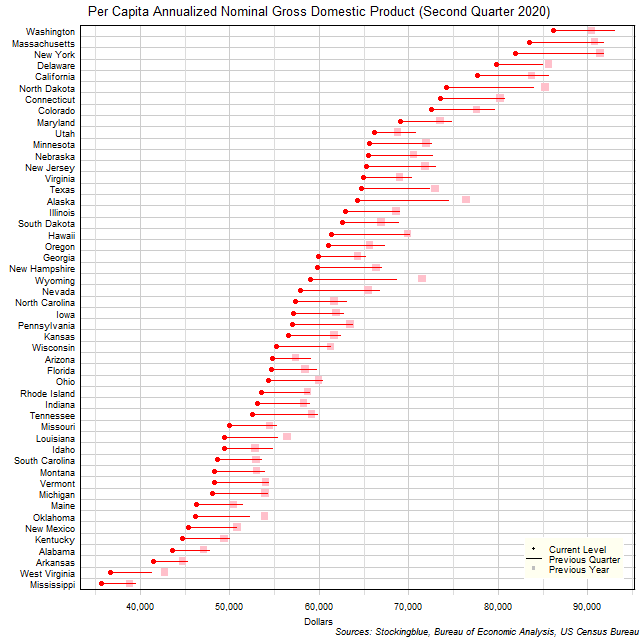The chart above shows the per capita annualized nominal gross domestic product (GDP) in each US state as of the second quarter of 2020 in dollars, the change from the previous quarter, and the GDP one year prior.  Every single state's economy contracted over the past quarter and the past year.

# EU and US GDP by Region, Second Quarter 2020

Oct 21, 2020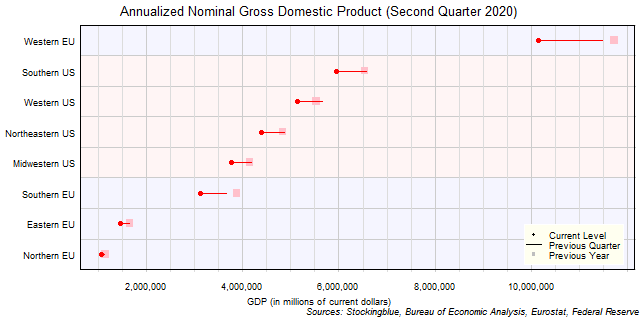The chart above shows the annualized nominal gross domestic product (GDP) in each EU and US region as of the second quarter of 2020 in millions of dollars, the change from the previous quarter, and the GDP one year prior.  Every single region's economy contracted over the past quarter and the past year.

# EU GDP by Region, Second Quarter 2020

Oct 20, 2020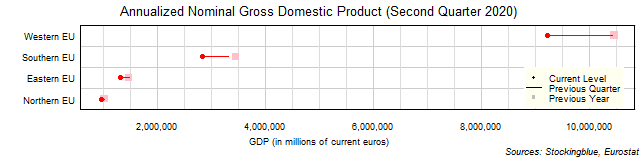The chart above shows the annualized nominal gross domestic product (GDP) in each EU region as of the second quarter of 2020 in millions of euros, the change from the previous quarter, and the GDP one year prior.  Every single region's economy contracted over the past quarter and the past year.

# US GDP by Region, Second Quarter 2020

Oct 19, 2020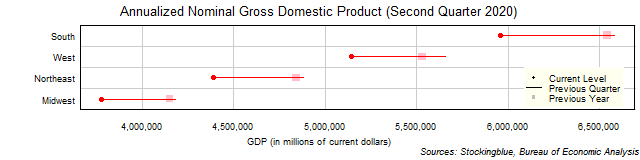The chart above shows the annualized nominal gross domestic product (GDP) in each US region as of the second quarter of 2020 in millions of dollars, the change from the previous quarter, and the GDP one year prior.  Every single region's economy contracted over the past quarter and the past year.

# EU and US GDP by State, Second Quarter 2020

Oct 16, 2020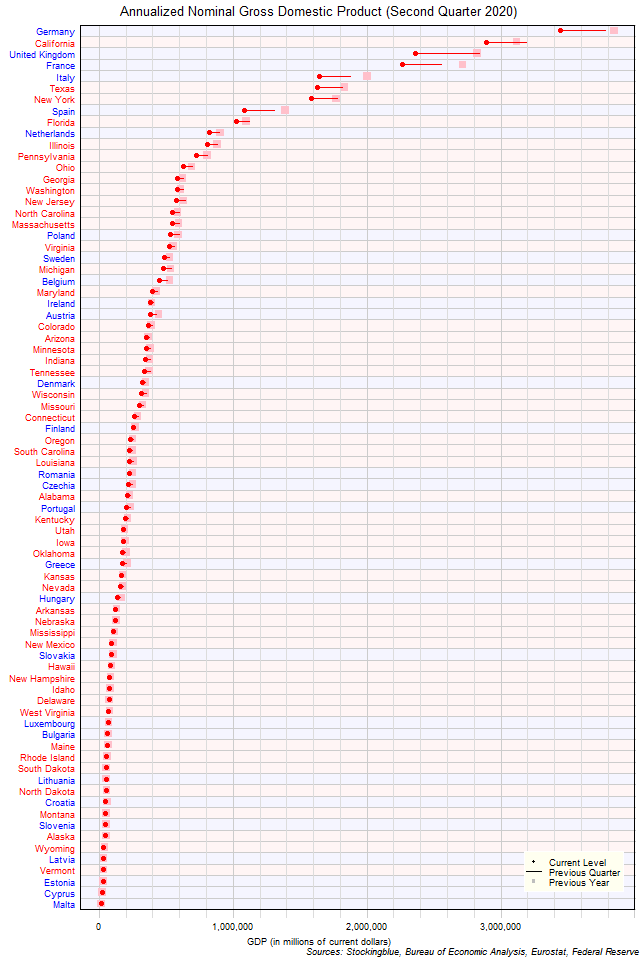The chart above shows the annualized nominal gross domestic product (GDP) in each EU and US state as of the second quarter of 2020 in millions of US dollars, the change from the previous quarter, and the GDP one year prior.  Every single state had a decrease in GDP over the previous quarter and over the previous year.

# EU GDP by State, Second Quarter 2020

Oct 15, 2020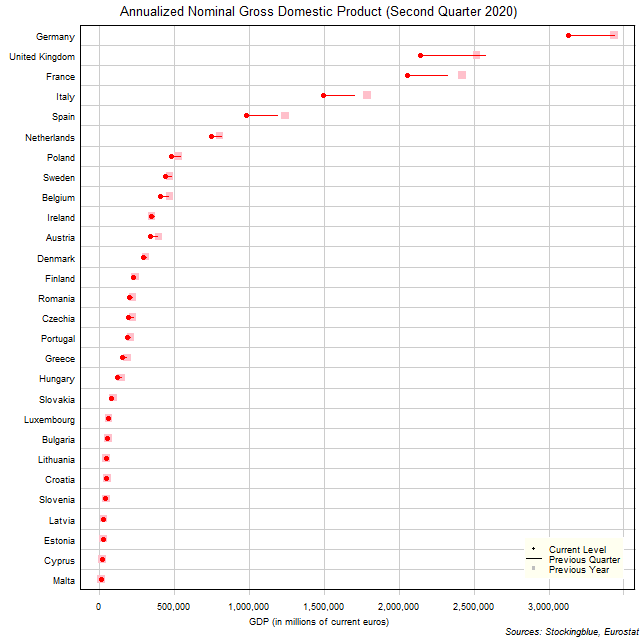The chart above shows the annualized nominal gross domestic product (GDP) in each EU state as of the second quarter of 2020 in millions of euros, the change from the previous quarter, and the GDP one year prior.  Every single state had a decrease in GDP over the previous quarter and over the previous year.

# US GDP by State, Second Quarter 2020

Oct 14, 2020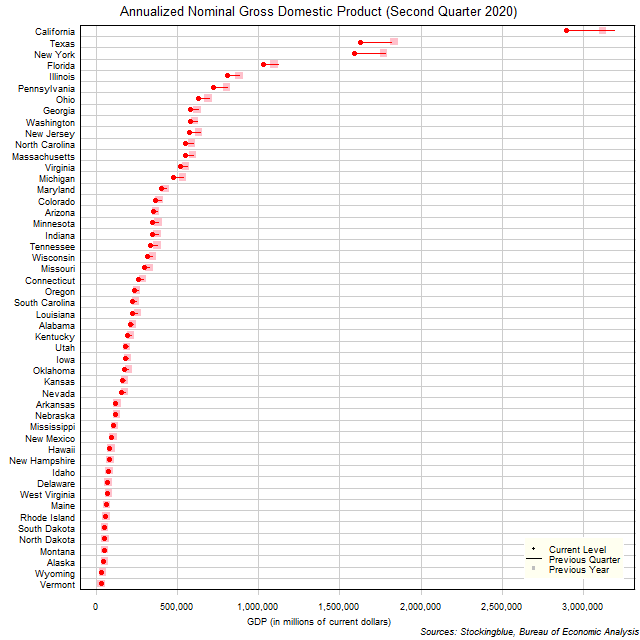The chart above shows the annualized nominal gross domestic product (GDP) in each US state as of the second quarter of 2020 in millions of dollars, the change from the previous quarter, and the GDP one year prior.  Every single state had a decrease in GDP over the previous quarter and over the previous year.

# Long-Term EU and US GDP Growth Rate by Region, First Quarter 2020

Sep 23, 2020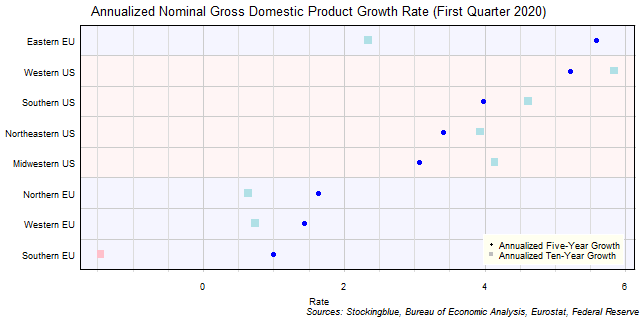The chart above shows the annualized nominal gross domestic product (GDP) growth rate when priced in US dollars in each EU and US region over the past five years as of the first quarter of 2020 and the growth over the past ten years.  All negative growth rates in the EU are attributed to currency rate fluctuations.

Older